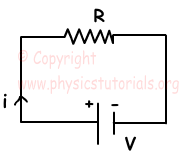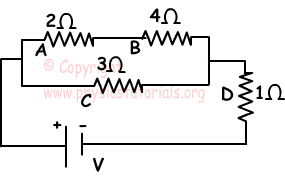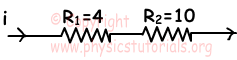# Electric Power and Energy with Examples

Electric Power and Energy

In a circuit given below, electrons coming from the battery transfer some of their energy to the conductor cable. They move and collide tothe particles of conductor and this transferred energy converted into heat energy. In a given unit of time we can find the emitted heat from the conductor as follows;

E=V.i.t

Where, E is the heat, V is the potential difference of the battery and t is the time. If we substitute i.R in terms of V then we find following energy equation.

E=i².R.t=V²/R.t

Electric Power

It is the energy emitted in a unit of time by the conductor.

Power=Electric Energy/time

If we substitute the energy formula, we get following equation for power.

P=V.i.t/t

P=i.V or if we put i.R in terms of V (ohm’s law)

P=i².R=V²/R

Unit of the power is watt

1 watt=joule/s

Example: Find the relation between the emitted energies by the resistors A, B, C and D in a unit of time.In this circuit, potential differences of the branches in parallel are equal. Currents passing through the branches are inversely proportional to the resistors. With these explanations, if the currents on the resistors A and B is i, then current on resistor C must be 2i.The sum of the currents 2i+i=3i coming from two branches pass through the last resistor D. Energy passing through a conductor in a unit of time is called power. Thus, powers of the resistors are;

From the equation P=i2.R

PA=i2.2Ω=2i2Ω

PB=i2.4Ω=4i2Ω

PC=(2i)2.3Ω=12i2Ω

PD=(3i)2.1Ω=9i2Ω

Relation between them is like;

PC>PD>PB>PA

Example: A radiator working with 30V potential difference has power 180 watt. Find the current passing through and resistance of the radiator.

We use the equation; P=i.V

180watt=i.30V

i=6 ampere

Resistance of the radiator R=V/i=30V/6A=5Ω

Example: If the current passing through this piece of circuit is i, power spent on the first resistance is 100watt. Find the potential difference between the ends of second resistor.P1=i².R1

100watt=i².4Ω

i=5Ampere

Potential difference between the ends of second resistor is,

V=i.R

V=5Ampere.10Ω=50Volt

Related